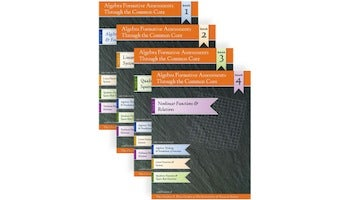Equity. Access. Excellence.

# Algebra Formative Assessments Through the Common Core series (Books, second edition, 2013)

## Algebra Formative Assessments Through the Common Core Series

### 4-volume setThis standards-aligned assessment series was developed for mathematics teachers and leaders with 121 tasks, aligned to the Common Core State Standards for Mathematics, grouped into four thematically related books.

Book 1: Algebra Formative Assessments Through the Common Core: Algebraic Thinking & Foundations of Functions presents 25 performance tasks aligned to selected Standards for Mathematical Content and Standards for Mathematical Practice. 250 pages.

Book 2: Algebra Formative Assessments Through the Common Core: Linear Functions & Systems presents 35 performance tasks aligned to selected Standards for Mathematical Content and Standards for Mathematical Practice. 304 pages.

Book 3: Algebra Formative Assessments Through the Common Core: Quadratic Functions & Square Root Functions presents 32 performance tasks aligned to selected Standards for Mathematical Content and Standards for Mathematical Practice. 330 pages.

Book 4: Algebra Formative Assessments Through the Common Core: Nonlinear Functions & Relations presents 29 performance tasks aligned to selected Standards for Mathematical Content and Standards for Mathematical Practice. 310 pages.

Each book provides alignment tables to help teachers select tasks and plan their use in the classroom. Each task is presented with the relevant Common Core State Standards for Mathematics alignment and with Teacher Notes that include guiding questions, model solutions, and extension questions. Selected tasks also include sample student work.